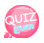# lambda function (anonymous function)

Today we will look at the lambda function.
Lambda functions are also called anonymous functions.
It's called anonymous.
Lambda functions do not have names.
In other words, it's called a closure.

## How to use lambda functions

```variable name = function(){
};```

It consists of the above structure.
We use lambda functions by assigning them to variables.
To call the lambda function, the variable name(); is.

## How to call a lambda function

```variable name = function(){
};

variable name();```

Now, let's use it.

```<?php
\$disney = function(){
echo "Hi I'm a lambda function";
};

\$disney();
?>```

Here is the result of the above code:You can also use parameters and arguments just like regular functions.

## Using parameter arguments in lambda functions

```variable name = function (\$param, \$param2){
};

variable name('arg','arg2');```

Then let's look at an example.

```<?php
\$disney = function(\$param){
echo \$param;
};

\$disney("Hi I'm a lambda function");
?>```

Here is the result of the above code: